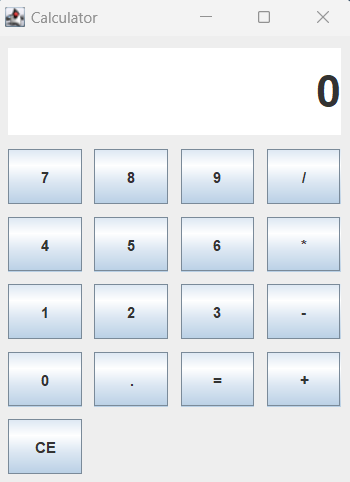# Create a Calculator in Java

In this tutorial, you will learn how to create a calculator in Java that looks like the one below:To create a calculator, we will use JFrame, JLabel, and JButton classes from the Swing framework. The JFrame class is used to construct a top-level window for a Java application.

Here's a code snippet that demonstrates how to create and display a calculator in Java:

```import java.awt.BorderLayout;
import java.awt.Color;
import java.awt.Dimension;
import java.awt.Font;
import java.awt.GridLayout;
import java.awt.event.ActionEvent;
import java.awt.event.ActionListener;
import java.util.Stack;
import javax.swing.BorderFactory;
import javax.swing.JButton;
import javax.swing.JFrame;
import javax.swing.JLabel;
import javax.swing.JPanel;
import javax.swing.SwingConstants;
import javax.swing.SwingUtilities;
import javax.swing.border.EmptyBorder;

public class Calculator {

private JLabel displayLabel;
private StringBuilder currentNumber;
private double result;

public Calculator() {
currentNumber = new StringBuilder();
result = 0.0;
}

private void createAndShowGUI() {
JFrame frame = new JFrame("Calculator");
frame.setDefaultCloseOperation(JFrame.EXIT_ON_CLOSE);
frame.setPreferredSize(new Dimension(300, 400));

JPanel mainPanel = new JPanel(new BorderLayout());
mainPanel.setBorder(BorderFactory.createEmptyBorder(10, 10, 10, 10));

JPanel displayPanel = new JPanel(new BorderLayout());
displayPanel.setPreferredSize(new Dimension(300, 80));
displayPanel.setBorder(new EmptyBorder(0, 0, 10, 0)); // Add bottom margin

displayLabel = new JLabel("0", SwingConstants.RIGHT);
displayLabel.setFont(new Font("Arial", Font.BOLD, 36));
displayLabel.setOpaque(true);
displayLabel.setBackground(Color.WHITE);

JPanel buttonPanel = new JPanel(new GridLayout(5, 4, 10, 10));

String[] buttonLabels = { "7", "8", "9", "/", "4", "5", "6", "*", "1", "2", "3", "-", "0", ".", "=", "+", "CE" };

for (String label : buttonLabels) {
JButton button = new JButton(label);
}

frame.pack();
frame.setLocationRelativeTo(null);
frame.setVisible(true);
}

private class ButtonClickListener implements ActionListener {
@Override
public void actionPerformed(ActionEvent e) {
String command = e.getActionCommand();

if (command.equals("=")) {
evaluateExpression();
} else if (command.equals("CE")) {
clearDisplay();
} else {
currentNumber.append(command);
displayLabel.setText(currentNumber.toString());
}
}

private void evaluateExpression() {
try {
result = evaluate(currentNumber.toString());
displayLabel.setText(String.valueOf(result));
currentNumber.setLength(0);
currentNumber.append(result);
} catch (Exception ex) {
displayLabel.setText("Error");
currentNumber.setLength(0);
}
}

private void clearDisplay() {
currentNumber.setLength(0);
displayLabel.setText("0");
}
}

public double evaluate(String expression) {
Stack operandStack = new Stack<>();
Stack operatorStack = new Stack<>();

for (int i = 0; i < expression.length(); i++) {
char ch = expression.charAt(i);

if (Character.isDigit(ch)) {
StringBuilder numBuilder = new StringBuilder();
while (i < expression.length() && (Character.isDigit(expression.charAt(i)) || expression.charAt(i) == '.')) {
numBuilder.append(expression.charAt(i));
i++;
}
i--;

double operand = Double.parseDouble(numBuilder.toString());
operandStack.push(operand);
} else if (ch == '(') {
operatorStack.push(ch);
} else if (ch == ')') {
while (!operatorStack.isEmpty() && operatorStack.peek() != '(') {
evaluateTopOperator(operandStack, operatorStack);
}
} else if (isOperator(ch)) {
while (!operatorStack.isEmpty() && precedence(operatorStack.peek()) >= precedence(ch)) {
evaluateTopOperator(operandStack, operatorStack);
}
operatorStack.push(ch);
}
}

while (!operatorStack.isEmpty()) {
evaluateTopOperator(operandStack, operatorStack);
}

return operandStack.pop();
}

private boolean isOperator(char ch) {
return ch == '+' || ch == '-' || ch == '*' || ch == '/';
}

private int precedence(char operator) {
if (operator == '+' || operator == '-') {
return 1;
} else if (operator == '*' || operator == '/') {
return 2;
}
return 0;
}

private void evaluateTopOperator(Stack operandStack, Stack operatorStack) {
double operand2 = operandStack.pop();
double operand1 = operandStack.pop();
char operator = operatorStack.pop();
double result = applyOperator(operand1, operand2, operator);
operandStack.push(result);
}

private double applyOperator(double operand1, double operand2, char operator) {
switch (operator) {
case '+':
return operand1 + operand2;
case '-':
return operand1 - operand2;
case '*':
return operand1 * operand2;
case '/':
return operand1 / operand2;
default:
throw new IllegalArgumentException("Invalid operator: " + operator);
}
}

public static void main(String[] args) {
SwingUtilities.invokeLater(() -> {
Calculator calculator = new Calculator();
calculator.createAndShowGUI();
});
}
}
```

The example code imports various classes from the java.awt and javax.swing packages that are necessary for creating the GUI. The class is named Calculator, which serves as the main class for the program. The class has instance variables, including a JLabel for displaying the numbers and results, a StringBuilder to store the current number being entered, and a double variable to hold the final result. The Calculator class has a constructor that initializes the instance variables. The createAndShowGUI method sets up the graphical user interface. It creates a JFrame window, sets its properties, and adds different panels and components to it. The displayPanel is a panel that contains a JLabel used to display the numbers and results. The buttonPanel is a panel that contains JButton components representing the buttons on the calculator. The ButtonClickListener class is an ActionListener that listens for button clicks. It performs actions based on the button clicked. For numeric buttons, it appends the corresponding number to the currentNumber StringBuilder and updates the display. For the "=" button, it evaluates the expression entered so far. For the "CE" button, it clears the display. The evaluateExpression method evaluates the arithmetic expression entered and updates the display with the result. It uses the evaluate method to perform the actual evaluation. The evaluate method uses two stacks, one for operands (numbers) and another for operators (+, -, *, /). It scans the expression character by character and performs the necessary calculations based on the operators and operands encountered. It follows the rules of operator precedence and uses the applyOperator method to perform the arithmetic operations. The isOperator method checks if a character is one of the four basic arithmetic operators. The precedence method assigns a precedence value to each operator, with higher values indicating higher precedence. The evaluateTopOperator method takes the top operator from the operator stack and applies it to the top two operands from the operand stack. It then pushes the result back onto the operand stack. The applyOperator method performs the actual arithmetic operation based on the given operator and operands. The main method is the entry point of the program. It creates an instance of the Calculator class and invokes the createAndShowGUI method to display the calculator window. Overall, this program creates a calculator GUI that allows users to enter arithmetic expressions and performs the calculations based on the input.